## Friday, October 12, 2018

### Unizor - Physics4Teens - Mechanics - Work - Golden Rule of Mechanics

Notes to a video lecture on http://www.unizor.com

Golden Rule of Mechanics

Let's summarize what we have learned about mechanical work in the previous lecture.

1. In a simple case of motion along a straight line with a constant force F acting along a trajectory, the most important parameter that quantifies the result of the action is work defined as W=F·S, that fully characterizes and is fully characterized by speed V of an object:

W = F·S = m·V²/2

In particular, it means that increasing the force by a factor of N and decreasing the distance it acts by the same factor of N would result in the same final speed of an object.

So, we can "win" in distance, but we will "lose" in force and vice versa.

This is the first example of the Golden Rule of Mechanics - there
are many ways to achieve the result, you can reduce your distance, but
you will have to increase the force or you can reduce the force, but you
will have to increase the distance.

In short, as we mentioned above, whatever you win in distance you lose in force and vice versa.

2. In case of a constant force acting at an angle to a straight line trajectory, the difference is only a factor cos(φ), where φ is an angle between a force and a direction of a trajectory. In vector form it represents the scalar product (F·S).

So, the Golden Rule works exactly as above.

3. Recall the formula for work of a force F pushing an object of weight P up along an inclined plane of the length S making angle φ with horizon to the height H:

W = F·S = P·H

We've proven this in the previous lecture and, as you see, it's
independent of the slope of an inclined plane. However, the minimum
effort we have to apply as a force to move an object up the slope is F=P·sin(φ), while the distance equals to S=H/sin(φ).

We can reduce the effort (the force F) by using an incline of a smaller slope, but that would lengthen the distance S we have to push an object.

So, again, we see the Golden Rule in action.

4. Consider lifting some heavy object of the weight P using a lever, applying the force F to the opposite to an object end of the lever.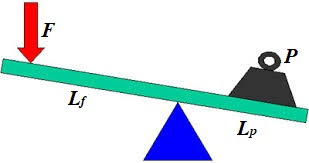This is a case of equilibrium in rotational motion and the balance can
be achieved by equating the moments of two forces acting against each
other:

F·Lf = P·Lp

If Sf is the distance the force F acts down and Sp is the distance our object moves up,

Sf/Sp = Lf/Lp and

F·Sf = P·Sp

By using a lever with longer arm Lf for the application of force, we can proportionally reduce the force F
achieving the same result - lifting an object to certain height. An
inverse is true as well - we can shorten the arm and proportionally
increase the force.

In any case, the Golden Rule of Mechanics is observed: "winning"
in force - proportionally "losing" in distance or "winning" in distance"
- proportionally "losing" in force.

All the above examples emphasize the importance of the concept of mechanical work as the quantitative measure and characteristic of the purpose and the result of applying a force. The so-called Golden Rule of Mechanics is just a catchy term that underscores the importance of the concept of work.

Acting with the force to achieve certain goal necessitates performing
certain amount of work that depends on the goal, not on how we achieve
it
.

## Wednesday, October 10, 2018

### Unizor - Physics4Teens - Mechanics - Work - Definition

Notes to a video lecture on http://www.unizor.com

Definition of Mechanical Work

Why do we apply a force to an object?

Usually, to achieve certain result, like to accelerate it to a certain speed or to move it from one point to another.

Straight line motion with a force acting along a trajectory

Consider an acceleration of a car by pressing the gas pedal. As a
result, a car will reach certain speed and then, in absence of the
traffic lights, disregarding friction and air resistance, it goes by
inertia, maintaining this constant speed.

A car with a more powerful engine will need a shorter distance to reach
certain speed. A car with a weaker engine accelerates on a longer
distance to reach the same speed.

It seems, there is some relationship between the force, the distance it
is applied to an object and the final speed reached by this object as a
result of the acting force.

Let's consider the final speed of a constant force F acting on an object of mass m, initially at rest, acting on certain distance S.

The acceleration of this object, according to the Second Newton's Law is

a = F/m

The time t to cover distance S with acceleration a is based on the formula of Kinematics

S = a·t²/2 and is equal to

t = √2S/a  = √2S·m/F

From this we derive the final speed at the end of acceleration

V = a·t = (F/m)·√2S·m/F  =

= √2F·S/m

As we see, the final speed of an object of mass m depends on the product of the force and the distance this force is acting.

This product that characterizes the result of applying a force on a certain distance is called the work performed by a given force acting on a given distance:

W = F·S

We can reduce by half the force and double the distance - the resulting speed will be the same.

Using this definition of work, we can represent the final speed as

V = √2W/m

Resolving for work as a function of final speed, we obtain

W = m·V²/2

So, given a final speed, we can determine how much work it takes to
achieve it and, given amount of work, we can calculate the speed this
work will cause.

This concept of work is closely tied with such less precisely
defined concepts of result, purpose, effect etc. While we not always can
compare the results or effect of two physical experiments, we can
always compare the work done by the forces participating in these experiments.

Thus, the work can be used to measure the results or effect of physical experiment.

Straight line motion with a force acting at an angle to a trajectory

Consider a slightly more complicated example with force acting at an angle to a trajectory.

A toy train of mass m is pulled by a child, who stands on a side of a track, with a constant force F, so the force acts at an angle to a straight line trajectory, making an angle φ with it.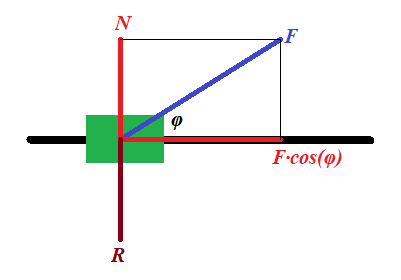Representing this force F (in blue on a picture above) as a
sum of two forces (in red), one acting along a track, forcing the train
to speed up, and another acting perpendicularly to a track (force N), which is balanced by the reaction of the track R (in brown), we see that the force that pushes a train forward equals to F·cos(φ), while the force acting perpendicularly to a track can be simply ignored as being balanced by an opposite reaction force.

Exactly the same considerations as above leads us to a more universal formula for relationship between the final speed and the work:

V = √2W/m ,

where W = F·S·cos(φ)

Obviously, if the force acts along a straight line of a trajectory, angle φ is zero, its cosine is 1 and the formula corresponds to the one derived earlier.

Resolving the formula above for work, we obtain

W = m·V²/2

which indicates that the work depends on the result of an action
only (final speed), not the way how we achieve this result, using a
stronger force on a shorter distance or a weaker force on a longer
distance.

Motion against gravity along an inclined plane

Let's consider a completely different goal of a physical experiment and show the importance of a concept of work.

This time our purpose is to lift an object of mass m to a certain height H above the ground along a frictionless inclined plane making angle φ with horizon.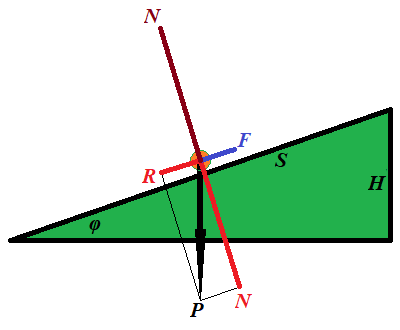The force F needed for this must be equal to a component R of the weight P of an object along the inclined because the other component of its weight N, perpendicular to an inclined, is balanced by a reaction of the plane.

F = P·sin(φ)

The distance S this force is acting on equals to

S = H/sin(φ)

From this we can derive the work performed by force F along the distance S:

W = F·S = P·sin(φ)·H/sin(φ) =

= P·H

This is quite a remarkable result. The work does not depend on the angle of an incline, only on the height we lift the object and its weight.

As in the previous cases, the work seems to be a characteristic
of the result and does not depend on how we have achieved it, using an
inclined with bigger or smaller slope.

Rotational motion with a force acting tangentially to a trajectory

Our final example is about rotation.

Consider a person starts rotating a carousel of a radius r, having a moment of inertia I, from the state of rest to some angular speed ω with constant angular acceleration α, applying constant force F tangentially to the carousel's rim.

From the rotational dynamics we can determine angular acceleration, knowing torque τ of the force and a moment of inertia of a carousel I:

τ = F·r = I·α

α = F·r/I

Linear acceleration of the point of application of the force equals to r·α.

To achieve the final angular speed ω with angular acceleration α we need time

t = ω/α = ω·I/(F·r)

During this time the point of application of force travels around a circle for a distance of

S = r·α·t²/2 =

= r·(F·r/I)·[ω·I/(F·r)]²/2 =

= I·ω²/(2F)

Multiplying by F both sides, we obtain the relationship between work W=F·S and final angular speed achieved as a result of application of the force:

W = F·S = I·ω²/2

This is a rotational equivalent of the analogous formula for straight line movement derived in the beginning of this lecture.

So, given a final angular speed, we can determine how much work it takes
to achieve it and, given amount of work, we can calculate the angular
speed this work will cause.

Definition of work in simple cases presented above

All the above examples provide a proper basis for the following definition of the work:

Work of the force F acting at an angle φ to trajectory on the distance S is W = F·S·cos(φ).

General case of a motion along a curved trajectory

To be more precise and to cover a case of a curved trajectory, we have
to require that this definition should be applied to infinitesimal
amount of work dW performed on infinitesimal interval dS of trajectory:

dW = F·dS·cos(φ)

Using a concept of scalar product of vectors and considering force and interval as vectors, the same definition can be written as

dW = (·d)

The latter represents the most rigorous definition of work.

## Wednesday, October 3, 2018

### Unizor - Physics4Teens - Mechanics - Statics - Problems

Notes to a video lecture on http://www.unizor.com

Problems on Equilibrium

Problem 1

Two point-objects of mass M and m are hanging at two opposite ends of a weightless rod of length L.

At what point on a rod should we fix a thread, so the system of a rod with two weights is hanging on this thread in equilibrium?

Does this state of equilibrium imply that a rod is horizontal?

At distance m·L /(M+m) from the end with object of mass M.

Problem 2

An object of weight W is positioned on a flat surface. The coefficient of static friction is μ.

What is the minimum pulling force P to be applied to a
rope, attached to this object, to start pulling it forward, if the angle
between a rope and a horizontal flat surface is φ?

P = μ·W / [cos(φ)+μ·sin(φ)]

Problem 3

Consider the following illustration to this problem.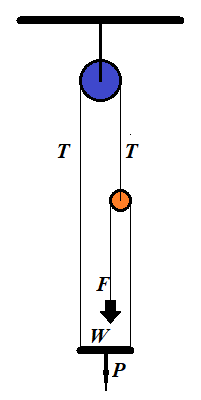A person of weight W stands on a platform of weight P. Pulleys and ropes are arranged as on this illustration.

What is the force F a person should apply to a rope to keep the whole system in equilibrium?

Solution

Let N be a reaction of a platform onto a person. It pushes the person upward.

If F is a force of a person pulling a rope down, and the
system is in equilibrium, that is all components are at rest and all
forces are balanced, the rope pulls a person up with the same force F, according to the Third Newton's Law.

Since a person is in equilibrium, forces directed upward (N+F) should be equal in magnitude to forces directed downwards (weight of the person W):

N+F = W

The platform is also in equilibrium. The forces that push it down are its weight P and reaction N of a person standing on it. The forces pulling the platform up are tensions of two ropes T on the left and F on the right:

P+N = T+F

Finally, a small pulley is in equilibrium. It's pulled down by two tensions F, from the left and from the right. The tension T pulls it up:

T = 2F

We have three equations with three unknowns: T, N and F, which we have to solve for an unknown force F, with which a person pulls a rope to balance the system:

Substituting T from the last equation into the second one, getting a system of two equations:

N+F = W

P+N = 3F

Solving the first equation for N and substituting into the second:

P+W−F = 3F

Resolving for F:

F = (P+W)/4

F = (P+W)/4

Problem 4

A ladder is standing at some angle to the floor. It's in equilibrium,
that is it's not slipping down to the floor. What holds it is the static friction between it and the floor.

If the ladder stands almost vertically, the static friction is
greater and it easily holds the ladder in the position. As we move the
bottom of a ladder away from the vertical, there will be a point when static friction will no longer could hold the ladder in place and the ladder would slip down to the floor.

What is the smallest angle the ladder would hold if the coefficient of static friction is μ?

Solution

Consider the following illustration to this problem.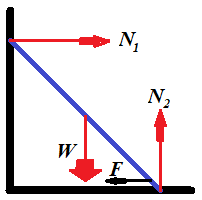While the reaction of the wall N1 is smaller than the static friction F, the ladder would hold its position.

The smallest angle between the ladder and the floor will be when these two forces are equal.

To be in equilibrium, vector sum of all forces must be equal to
null-vector and a sum of all angular momentums also must be equal to
null-vector.

Along the horizontal X-axis the sum of all forces equals to zero if the horizontal reaction of the wall N1 equals to the force of static friction F, which, in turn, equals to a product of the vertical reaction of the floor N2 by a coefficient of static friction μ:

(a) N1 = μ·N2

Along the vertical Y-axis the sum of forces equals to zero if the reaction of the floor N2 equals to weight of a ladder W:

(b) N2 = W

To analyze angular momentums, let's choose an axis of rotation. It's
easier to choose an axis perpendicular to both X- and Y-axis that goes
through a point where a ladder touches the floor. In this case angular
momentums of forces N2 and F are zero because their corresponding radiuses are zero.

Let's assume that the length of the ladder is D and the angle between the ladder and the floor is φ. The magnitude of the angular momentum of the force of weight W will then be W·D·cos(φ)/2. The magnitude of the angular momentum of the reaction of the wall N1 will be N1·D·sin(φ). The condition of the sum of angular momentums to be equal to zero results in the equation:

N1·D·sin(φ) = W·D·cos(φ)/2

The length of the ladder can be canceled:

(c) N1·sin(φ) = W·cos(φ)/2

Equations (a), (b) and (c) form a system of three equations with three unknowns:

(a) N1 = μ·N2

(b) N2 = W

(c) N1·sin(φ) = W·cos(φ)/2

From this

tan(φ) = 1/(2μ)